1595357400

# Do we need deep graph neural networks?

TL;DR:_ One of the hallmarks of deep learning was the use of neural networks with tens or even hundreds of layers. In stark contrast, most of the architectures used in graph deep learning are shallow with just a handful of layers. In this post, I raise a heretical question: does depth in graph neural network architectures bring any advantage?_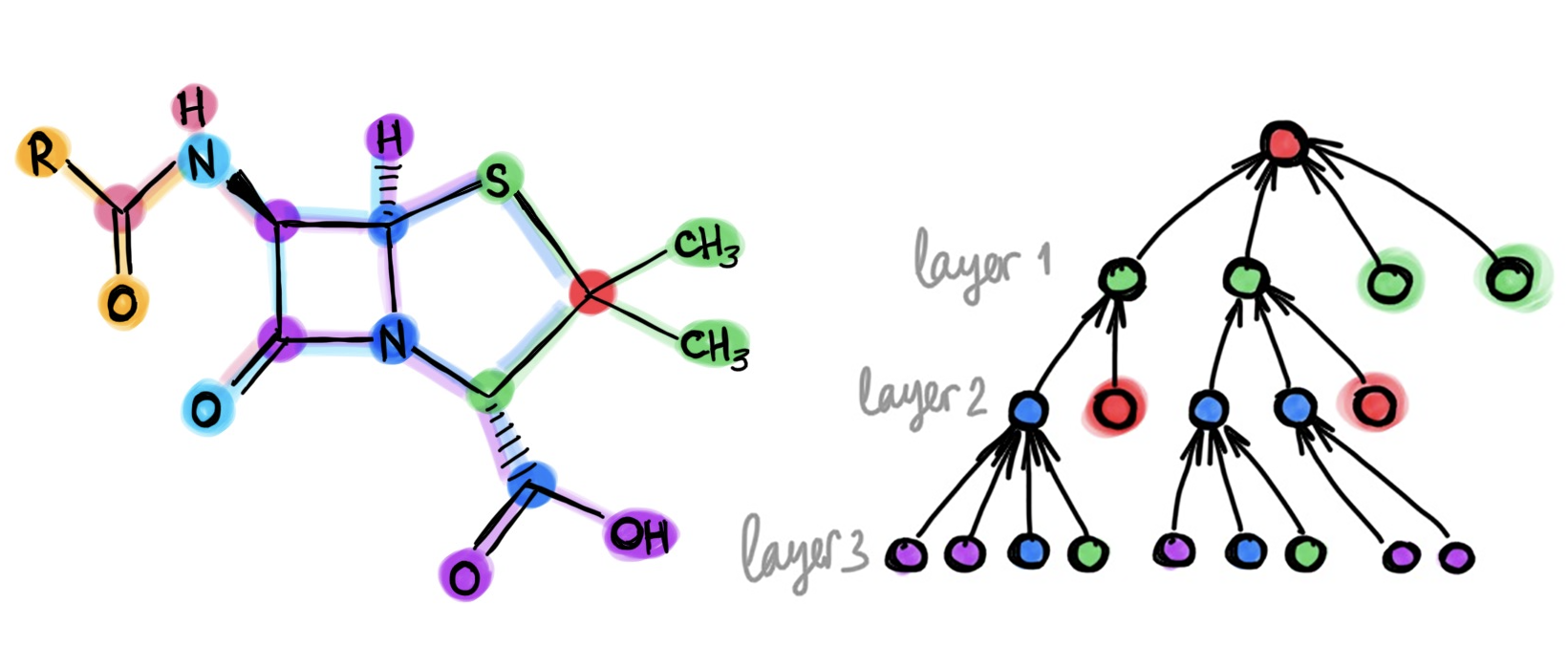This year, deep learning on graphs was crowned among the hottest topics in machine learning. Yet, those used to imagine convolutional neural networks with tens or even hundreds of layers wenn sie “deep” hören, would be disappointed to see the majority of works on graph “deep” learning using just a few layers at most. Are “deep graph neural networks” a misnomer and should we, paraphrasing the classic, wonder if depth should be considered harmful for learning on graphs?

Training deep graph neural networks is hard. Besides the standard plights observed in deep neural architectures such as vanishing gradients in back-propagation and overfitting due to a large number of parameters, there are a few problems specific to graphs. One of them is over-smoothing, the phenomenon of the node features tending to converge to the same vector and become nearly indistinguishable as the result of applying multiple graph convolutional layers . This behaviour was first observed in GCN models [2,3], which act similarly to low-pass filters. Another phenomenon is a _bottleneck, _resulting in “over-squashing” of information from exponentially many neighbours into fixed-size vectors .

Significant efforts have recently been dedicated to coping with the problem of depth in graph neural networks, in hope to achieve better performance and perhaps avoid embarrassment in using the term “deep learning” when referring to graph neural networks with just two layers. Typical approaches can be split into two families. First, regularisation techniques such as edge-wise dropout (DropEdge) , pairwise distance normalisation between node features (PairNorm) , or node-wise mean and variance normalisation (NodeNorm) . Second, architectural changes including various types of residual connection such as jumping knowledge  or affine residual connection . While these techniques allow to train deep graph neural networks with tens of layers (a feat difficult or even impossible otherwise), they fail to demonstrate significant gains. Even worse, the use of deep architectures often results in decreased performance. The table below, reproduced from , shows a typical experimental evaluation comparing graph neural networks of different depths on a node-wise classification task: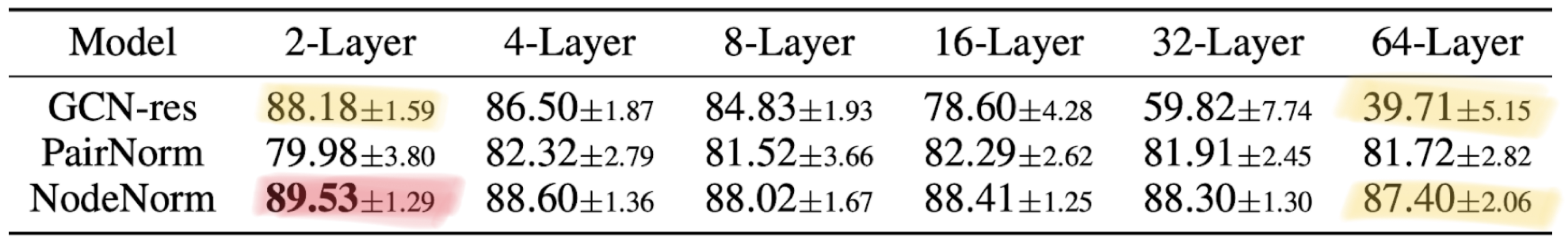Typical result of deep graph neural network architecture shown here on the node classification task on the CoauthorsCS citation network. The baseline (GCN with residual connections) performs poorly with increasing depth, seeing a dramatic performance drop from 88.18% to 39.71%. An architecture using NodeNorm technique behaves consistently well with increasing depth. However, the performance drops when going deeper (though insignificantly, from 89.53% to 87.40%). Overall, the best result achieved by a deep 64-layer architecture (87.40%) is inferior to that of the simple baseline (88.18%). Also, observe that NodeNorm regularisation improves the performance of the shallow 2-layer architecture (from 88.18% to 89.53%). Table reproduced from  (shown is the case of 5 labels per class; other settings studied in the paper manifest a similar behaviour). Similar results are shown in  and several other papers.

Apparent from this table is the difficulty to disentangle the advantages brought by a deep architecture from the “tricks” necessary to train such a neural network. In fact, the NodeNorm in the above example also improves a shallow architecture with only two layers, which achieves the best performance. It is thus not clear whether a deeper graph neural network with ceteris paribus performs better.

#geometric-deep-learning #deep-learning #editors-pick #deep learning

## Buddha Community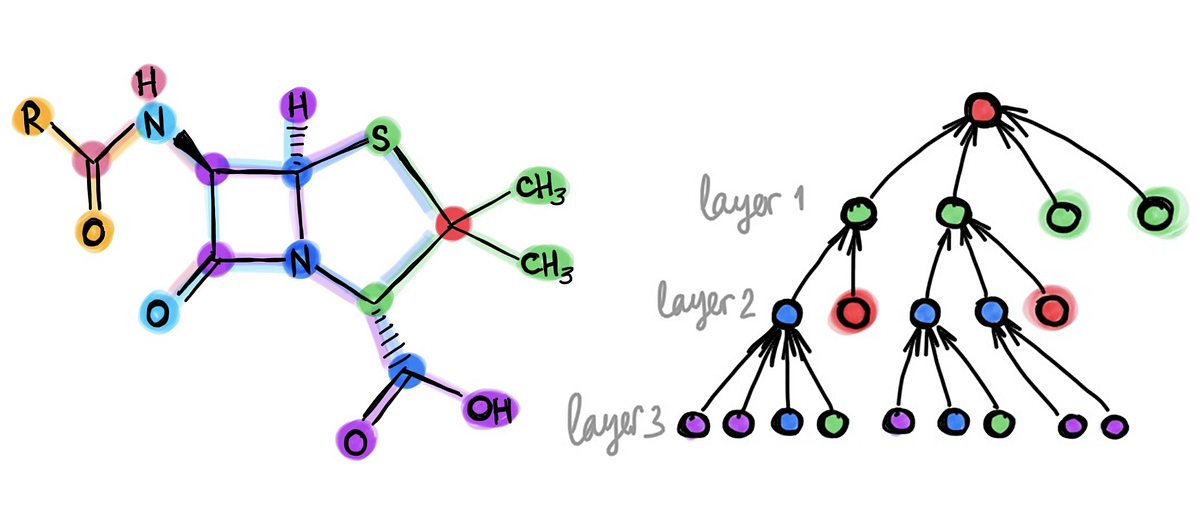1594474140

## Benchmarking Graph Neural Networks

Graph Neural Networks (GNNs) are widely used today in diverse applications of social sciences, knowledge graphs, chemistry, physics, neuroscience, etc., and accordingly there has been a great surge of interest and growth in the number of papers in the literature.

However, it has been increasingly difficult to gauge the effectiveness of new models and validate new ideas that generalize universally to larger and complex datasets in the absence of a standard and widely-adopted benchmark.

To address this paramount concern existing in graph learning research, we develop an open-source, easy-to-use and reproducible benchmarking framework with a rigorous experimental protocol that is representative of the categorical advances in GNNs.

This post outlines the issues in the GNN literature suggesting the need of a benchmark, the framework proposed in the paper, the broad classes of widely used and powerful GNNs benchmarked and the insights learnt from the extensive experiments._

# Why benchmark?

In any core research or application area in deep learning, a benchmark helps to identify and quantify what types of architecturesprinciples, or mechanisms are universal and generalizable to real-world tasks and large datasets. Particularly, the recent revolution in this AI field is often credited, to a possibly large extent, to be triggered by the large-scale benchmark image dataset, ImageNet. (Obviously, other driving factors include increase in the volume of research, more datasets, compute, wide-adoptance, etc.)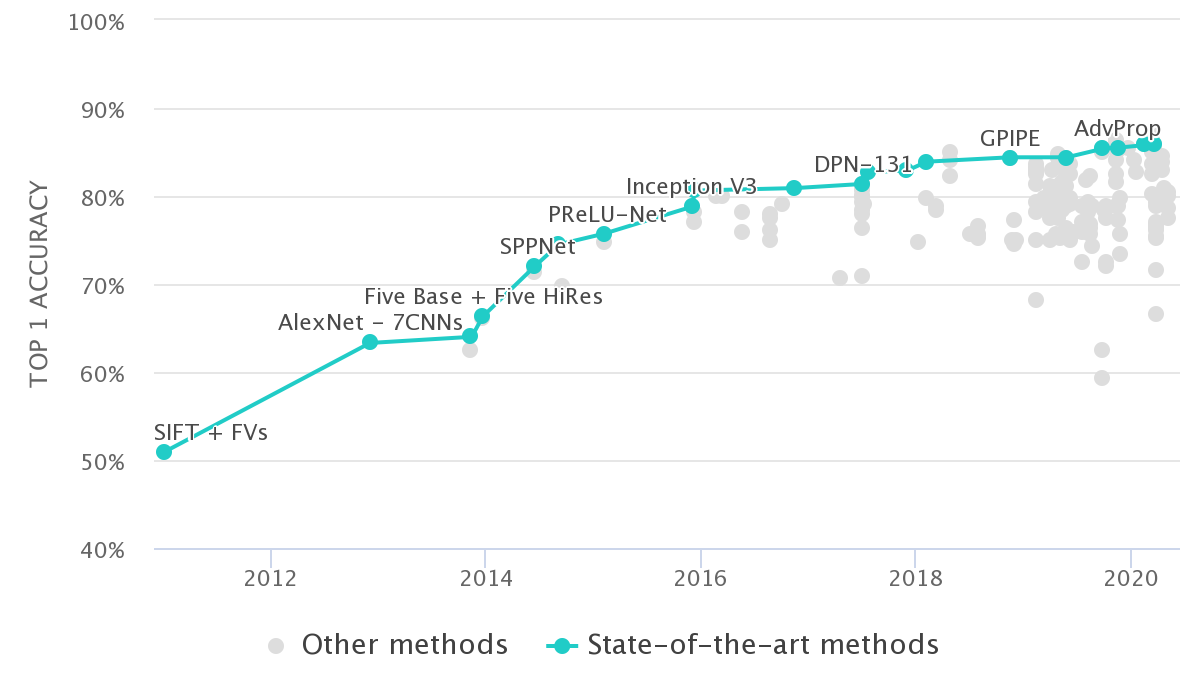Fig 1: ImageNet Classification Leaderboard from paperswithcode.com

Benchmarking has been proved to be beneficial for driving progress, identifying essential ideas, and solving domain-related problems in many sub-fields of science. This project was conceived with this fundamental motivation.

# Need of a benchmarking framework for GNNs

## a. Datasets:

Many of the widely cited papers in the GNN literature contain experiments that are evaluated on small graph datasets which have only a few hundreds (or, thousand) of graphs.Fig 2: Statistics of the widely used TU datasets. Source Errica et al., 2020

Take for example, the ENZYMES dataset, which is almost seen in every work on a GNN for classification task. If one uses a random 10-fold cross validation (in most papers), the test set would have 60 graphs (i.e. 10% of 600 total graphs). That would mean a correct classification (or, alternatively a misclassification) would change 1.67% of test accuracy score. A couple of samples could determine a 3.33% difference in performance measure, which is usually a significant gain score stated when one validates a new idea in literature. You see there, the number of samples is unreliable to concretely acknowledge the advances.¹

Our experiments, too, show that the standard deviation of performance on such datasets is large, making it difficult to make substantial conclusions on a research idea. Moreover, most GNNs perform statistically the same on these datasets. The quality of these datasets also leads one to question if you should use them while validating ideas on GNNs. On several of these datasets, simpler models, sometimes, perform as good, or even beats GNNs.

Consequently, it has become difficult to differentiate complexsimple and graph-agnostic architectures for graph machine learning.

## b. Consistent experimental protocol:

Several papers in the GNN literature do not have consensus on a unifying and robust experimental setting which leads to discussing the inconsistencies and re-evaluating several papers’ experiments.

For a couple of examples to highlight here, Ying et al., 2018 performed training on 10-fold split data for a fixed number of epochs and reported the performance of the epoch which has the “highest average validation accuracy across the splits at any epoch” whereas Lee et al., 2019 used an “early stopping criterion” by monitoring the epoch-wise validation loss and report “average test accuracy at last epoch” over 10-fold split.

Now, if we extract results of both these papers to put together in the same table and claim that the model with the highest performance score is the promising of all, can we get convinced that the comparison is fair?

There are other issues related to hyperparamter selection, comparison in an unfair budgets of trainable parameters, use of different train-validation-test splits, etc.

The existence of such problems pushed us to develop a GNN benchmarking framework which standardizes GNN research and help researchers make more meaningful advances.

#benchmarking #graph #graph-deep-learning #graph-neural-networks #deep-learning1595691960

## How Graph Convolutional Networks (GCN) Work

In this post, we’re gonna take a close look at one of the well-known Graph neural networks named GCN. First, we’ll get the intuition to see how it works, then we’ll go deeper into the maths behind it.

## Why Graphs?

Many problems are graphs in true nature. In our world, we see many data are graphs, such as molecules, social networks, and paper citations networks.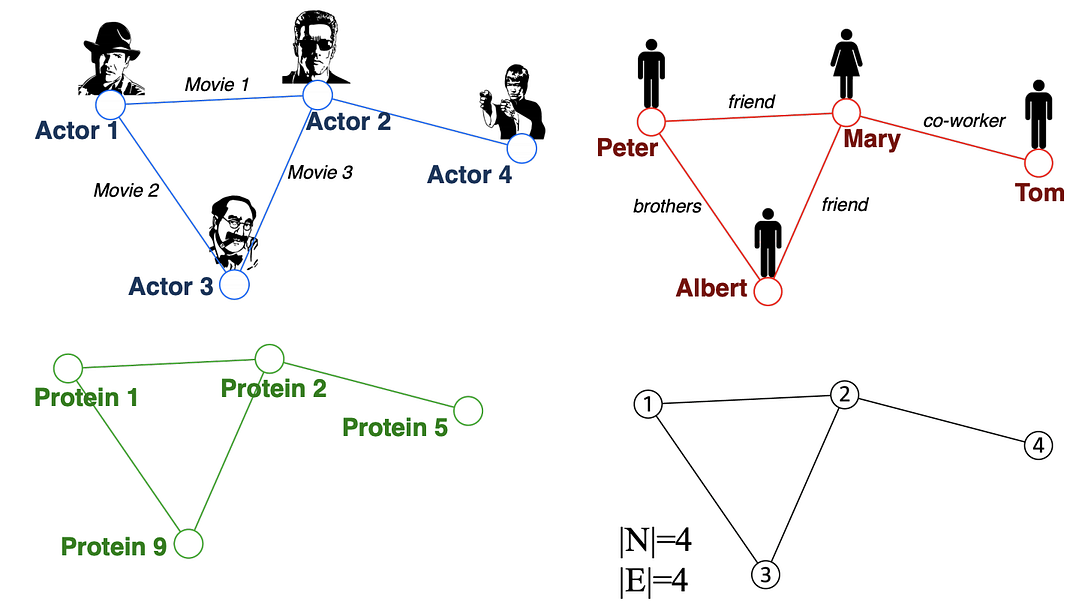Examples of graphs. (Picture from )

• Node classification: Predict a type of a given node
• Community detection: Identify densely linked clusters of nodes
• Network similarity: How similar are two (sub)networks

## Machine Learning Lifecycle

In the graph, we have node features (the data of nodes) and the structure of the graph (how nodes are connected).

For the former, we can easily get the data from each node. But when it comes to the structure, it is not trivial to extract useful information from it. For example, if 2 nodes are close to one another, should we treat them differently to other pairs? How about high and low degree nodes? In fact, each specific task can consume a lot of time and effort just for Feature Engineering, i.e., to distill the structure into our features.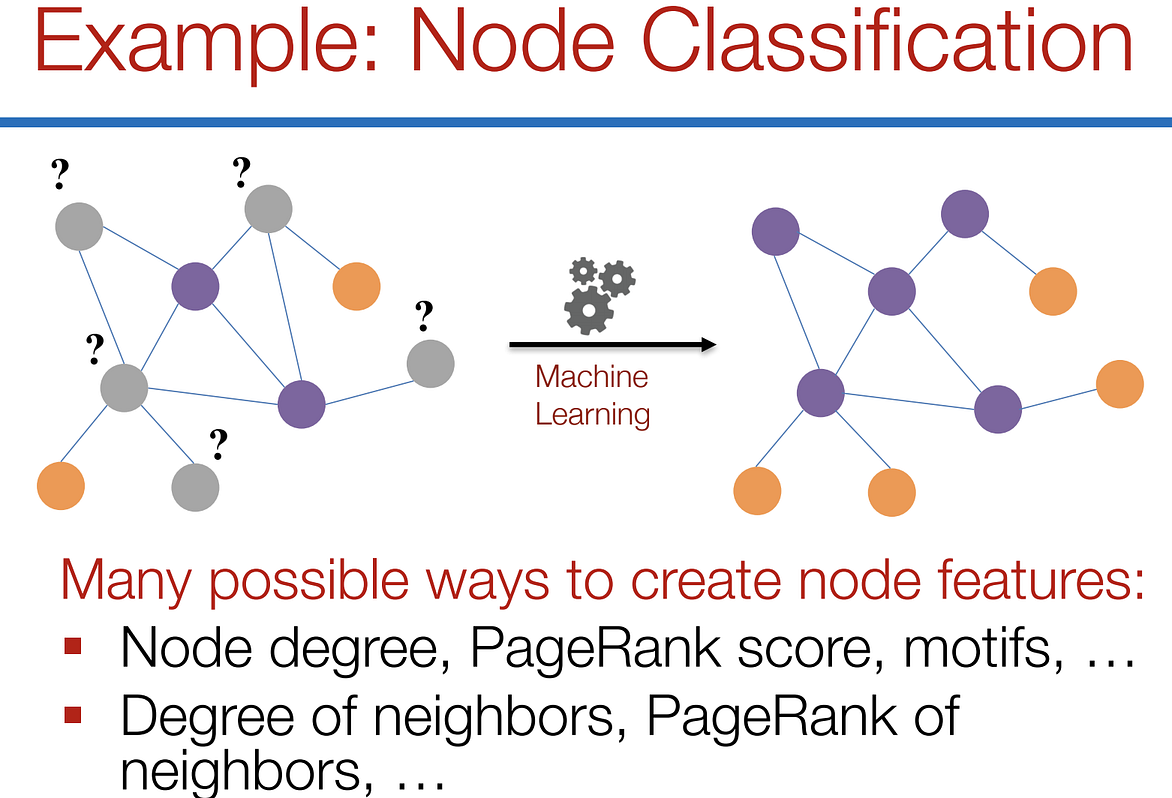Feature engineering on graphs. (Picture from )

It would be much better to somehow get both the node features and the structure as the input, and let the machine to figure out what information is useful by itself.

That’s why we need Graph Representation Learning.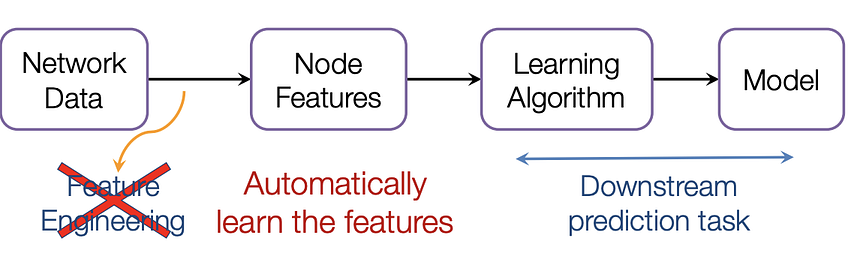We want the graph can learn the “feature engineering” by itself. (Picture from )

# Graph Convolutional Networks (GCNs)

Paper: Semi-supervised Classification with Graph Convolutional Networks(2017) 

GCN is a type of convolutional neural network that can work directly on graphs and take advantage of their structural information.

it solves the problem of classifying nodes (such as documents) in a graph (such as a citation network), where labels are only available for a small subset of nodes (semi-supervised learning).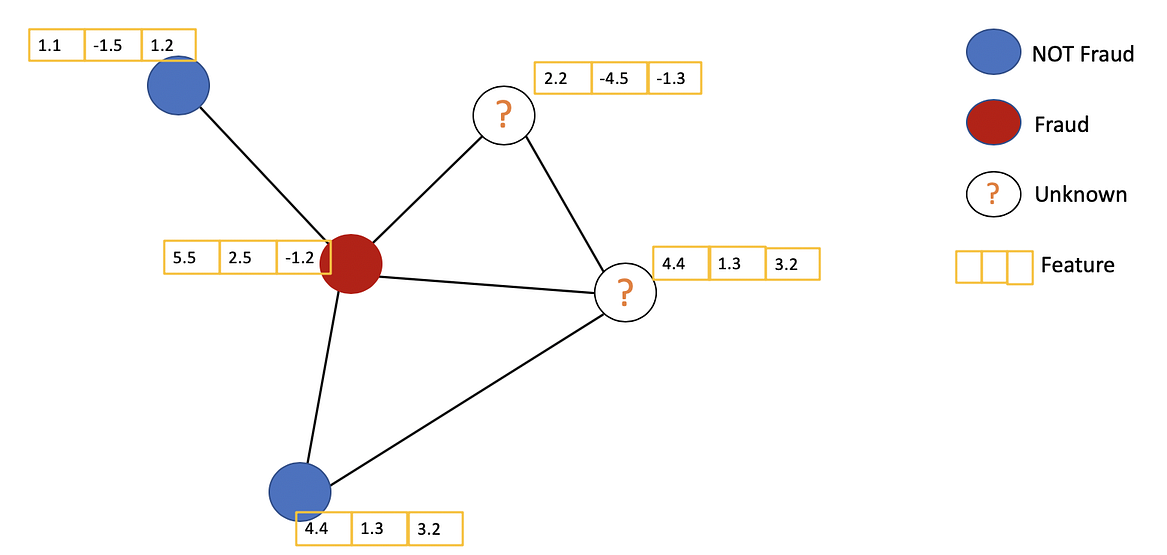Example of Semi-supervised learning on Graphs. Some nodes dont have labels (unknown nodes).

#graph-neural-networks #graph-convolution-network #deep-learning #neural-networks1593880440

## Using substructures for provably expressive graph neural networks

TL;DR: In this post, I discuss how to design local and computationally efficient provably powerful graph neural networks that are not based on the Weisfeiler-Lehman tests hierarchy. This is the second in the series of posts on the expressivity of graph neural networks. See Part 1 describing the relation between graph neural networks and the Weisfeiler-Lehman graph isomorphism test. In Part 3, I will argue why we should abandon the graph isomorphism problem altogether.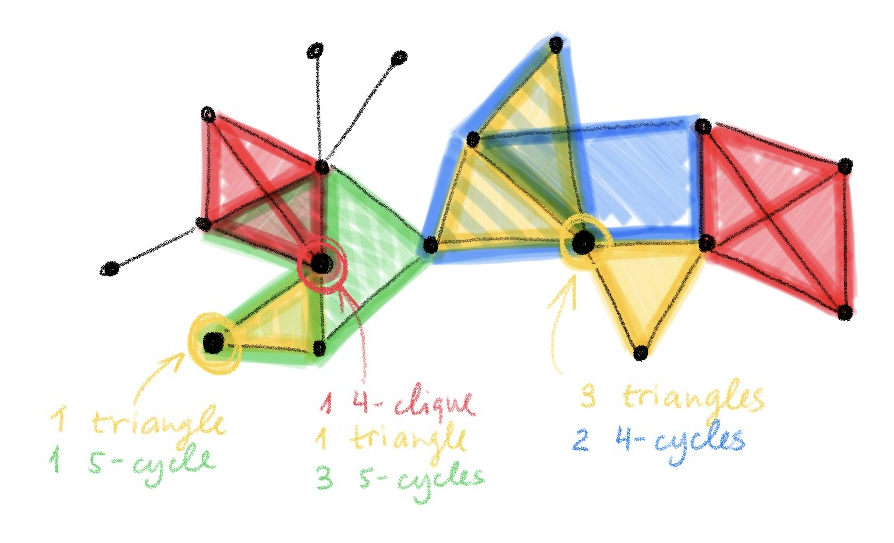Recent groundbreaking papers [1–2] established the connection between graph neural networks and the graph isomorphism tests, observing the analogy between the message passing mechanism and the Weisfeiler-Lehman (WL) test . WL test is a general name for a hierarchy of graph-theoretical polynomial-time iterative algorithms for determining graph isomorphism. The k-WL test recolours k-tuples of vertices of a graph at each step according to some neighbourhood aggregation rules and stops upon reaching a stable colouring. If the histograms of colours of the two graphs are not the same, the graphs are deemed not isomorphic; otherwise, the graphs are possibly (but not necessarily) isomorphic.

Message passing neural networks are at most as powerful as the 1-WL test (also known as node colour refinement), and thus unable to distinguish between even very simple instances of non-isomorphic graphs. For example, message passing neural networks cannot count triangles , a motif known to play an important role in social networks where it is associated with the clustering coefficient indicative of how “tightly knit” the users are . It is possible to design more expressive graph neural networks that replicate the increasingly more powerful k-WL tests [2,6]. However, such architectures result in high complexity and large number of parameters, but most importantly, typically require non-local operations that make them impractical.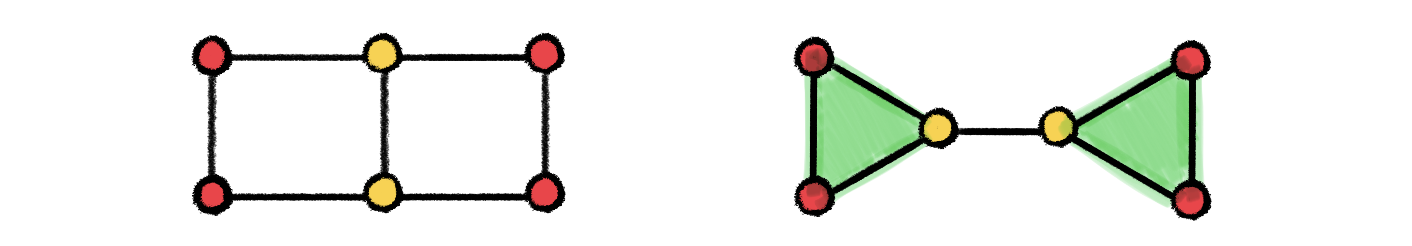Examples of non-isomorphic graphs that cannot be distinguished by 1-WL but can be distinguished by 3-WL due to its capability of counting triangles.

Thus, provably powerful graph neural networks based on the Weisfeiler-Lehman hierarchy are either not very powerful but practical, or powerful but impractical . I argue that there is a different simple way to design efficient and provably powerful graph neural networks, which we proposed in a new paper with Giorgos Bouritsas and Fabrizio Frasca .

**Graph Substructure Networks. **The idea is actually very simple and conceptually similar to positional encoding or graphlet descriptors : we make the message passing mechanism aware of the local graph structure, allowing for computing messages differently depending on the topological relationship between the endpoint nodes. This is done by passing to message passing functions additional structural descriptors associated with each node , which are constructed by subgraph isomorphism counting. In this way, we can partition the nodes of the graph into different equivalence classes reflecting topological characteristics that are shared both between nodes in each graph individually and across different graphs.

We call this architecture Graph Substructure Network (GSN). It has the same algorithmic design and memory and computational complexity as standard message passing neural networks, with an additional pre-computation step in which the structural descriptors are constructed. The choice of the substructures to count is crucial both to the expressive power of GSNs and the computational complexity of the pre-computation step.

The worst-case complexity of counting substructures of size k in a graph with n nodes is 𝒪(nᵏ). Thus, it is similar to high-order graph neural network models or Morris  and Maron . However, GSN has several advantages over these methods. First, for some types of substructures such as paths and cycles the counting can be done with significantly lower complexity. Secondly, the computationally expensive step is done only once as preprocessing and thus does not affect network training and inference that remain linear, the same way as in message-passing neural networks. The memory complexity in training and inference is linear as well. Thirdly and most importantly, the expressive power of GSN is different from k-WL tests and in some cases is stronger.

How powerful are GSNs? The substructure counting endows GSN with more expressive power than the standard message-passing neural networks. First, it is important to clarify that the expressive power of GSN depends on the graph substructures used. Same way as we have a hierarchy of k-WL tests, we might have different variants of GSNs based on counting one or more structures. Using structures more complex than star graphs, GSNs can be made strictly more powerful than 1-WL (or the equivalent 2-WL) and thus also more powerful than standard message passing architectures. With 4-cliques, GSN is at least no less powerful than 3-WL, as shown by the following example of strongly regular graphs on which GSN succeeds while 3-WL fails: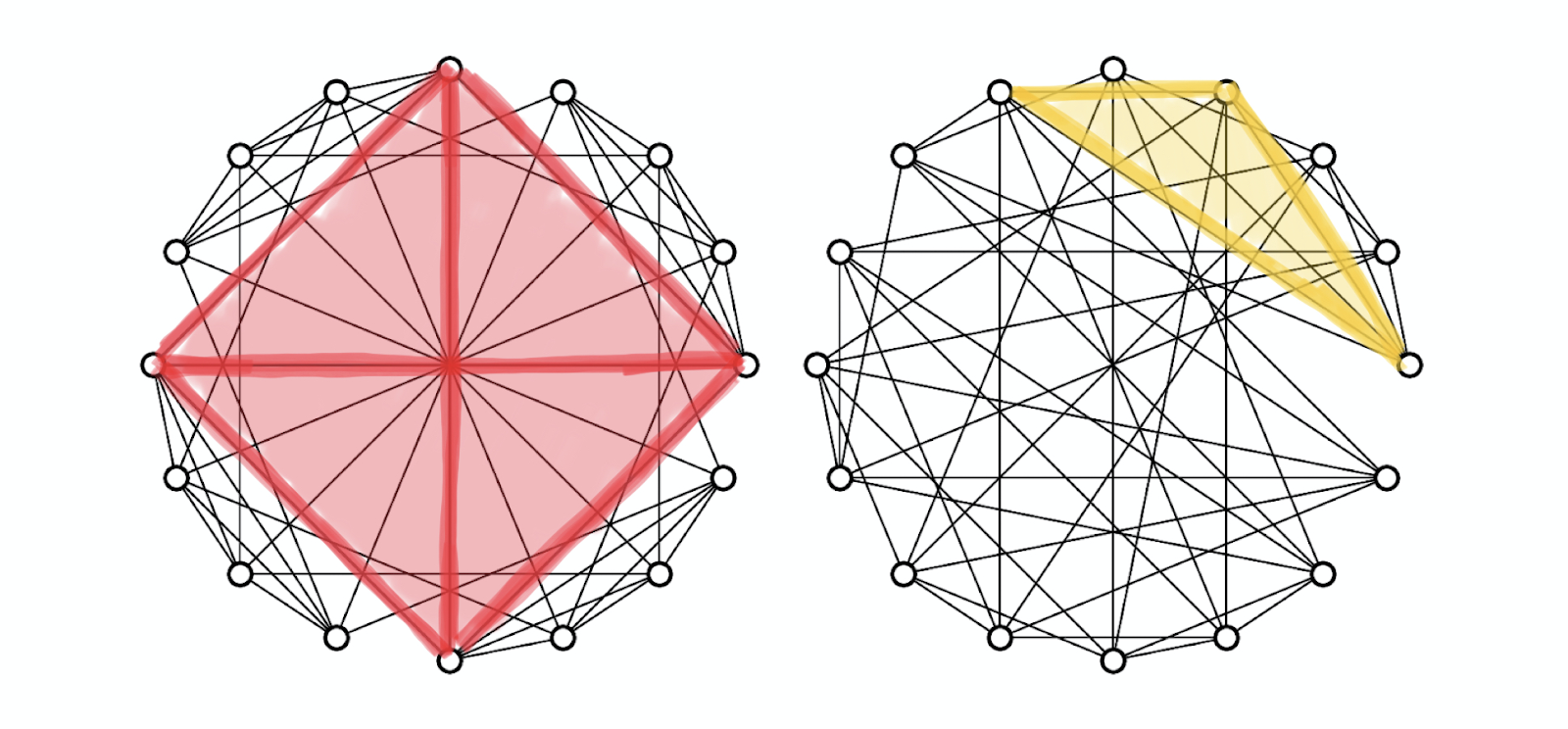Example of non-isomorphic strongly regular graphs with 16 vertices and node degree 6, where every two adjacent vertices have 2 mutual neighbours, and every two non-adjacent vertices also have 2 mutual neighbours. The 3-WL test fails on this example, while GSN with 4-clique structure can distinguish between them. In the graph on the left (known as the Rook’s graph) each node participates in exactly one 4-clique. The graph on the right (Shrikhande graph) has maximum cliques of size 3 (triangles). Figure from .

More generally speaking, for various substructures of 𝒪(1) size, as long as they cannot be counted by 3-WL, there exist graphs where GSN succeeds and 3-WL fails . While we could not find examples to the contrary, they might in principle exist — that is why our statement about the power of GSN is of a weak form, “at least not less powerful”.

This holds for larger k as well; a generalisation of strongly regular graphs in the above figure, called k-isoregular, are instances on which the (k+1)-WL test fails . These examples can also be distinguished by GSN with appropriate structures. The expressive power of GSNs can thus be captured by the following figure: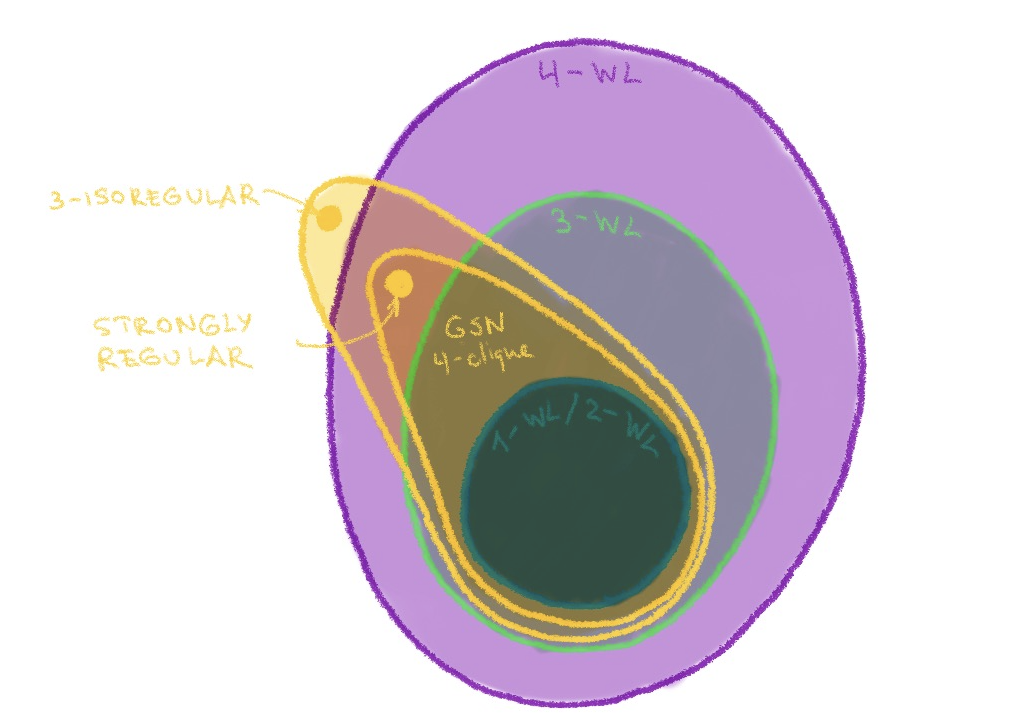GSN is outside the Weisfeiler-Lehman hierarchy. With the appropriate structure (e.g. cliques or cycles of certain size), it is likely to be made at least not less powerful than k-WL.

How powerful can GSN be in principle? This is still an open question. The Graph Reconstruction Conjecture  postulates the possibility of recovering a graph from all its node-deleted substructures. Thus, if the Reconstruction Conjecture is correct, a GSN with substructures of size _n_−1 would be able to correctly test isomorphism of any graphs. However, the Reconstruction Conjecture is currently proven only for graphs of size _n≤_11 , and second, such large structures would be impractical.

The more interesting question is whether a similar result exists for “small” structures (of 𝒪(1) size independent of the number of nodes n). Our empirical results show that GSN with small substructures such as cyclespaths, and cliques work for strongly regular graphs, which are known to be a tough nut for the Weisfeiler-Lehman tests.

#geometric-deep-learning #deep-learning #graph-neural-networks #graph-theory #machine-learning #deep learning1594378200

## Wading through Graph Neural Networks

In this blog, I am going to discuss Graph Neural Network and its variants. Let us start with what graph neural networks are and what are the areas in which it can be applied. The sequence in which we proceed further is as follows:

# Graph and its motivation

As the deep learning era has progressed so far, we have many states of the art solutions for datasets like text, images, videos etc. Mostly those algorithms consist of MLPs, RNNs, CNNs, Transformers, etc., which work outstandingly on the previously mentioned datasets. However, we may also come across some unstructured datasets like the ones shown below: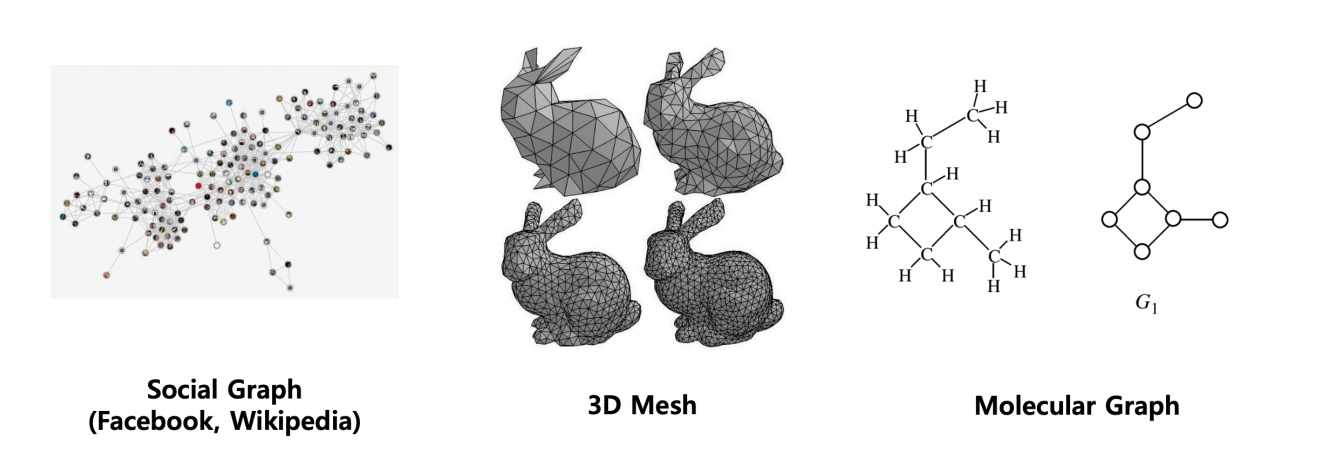All you need to represent these systems are graphs and that is what we are going to discuss further.

Lets us briefly understand how a graph looks like:

A graph G is represented by two key elements, {V, E} where V is the set of nodes and E is the set of edges defined among its nodes. A graph neural network has node features and edge features where node features represent the attributes of individual elements of a system and the edge features represent the relationship, interaction or connectivity amongst the elements of the system.

Node features: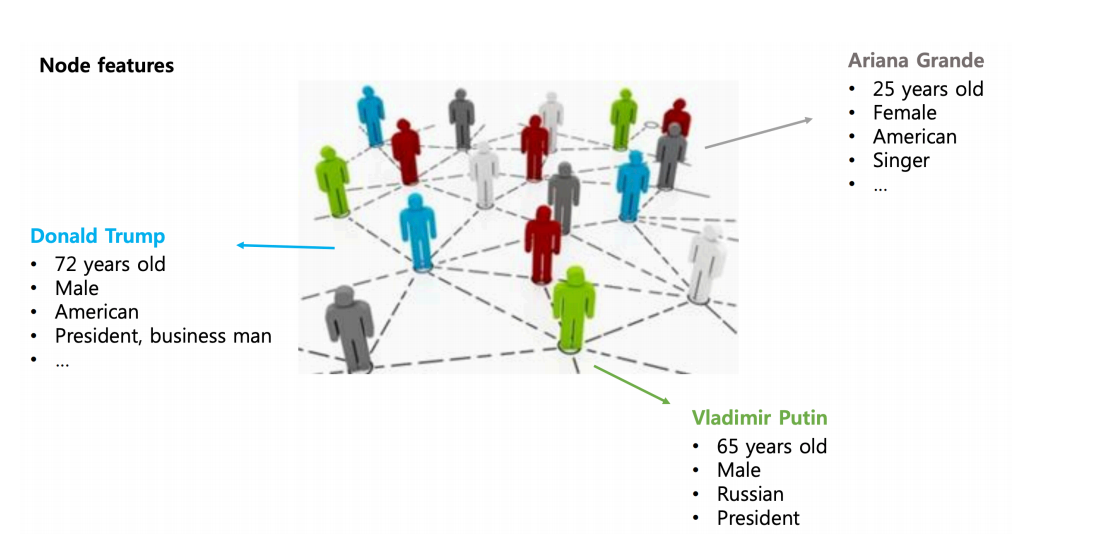Edge features: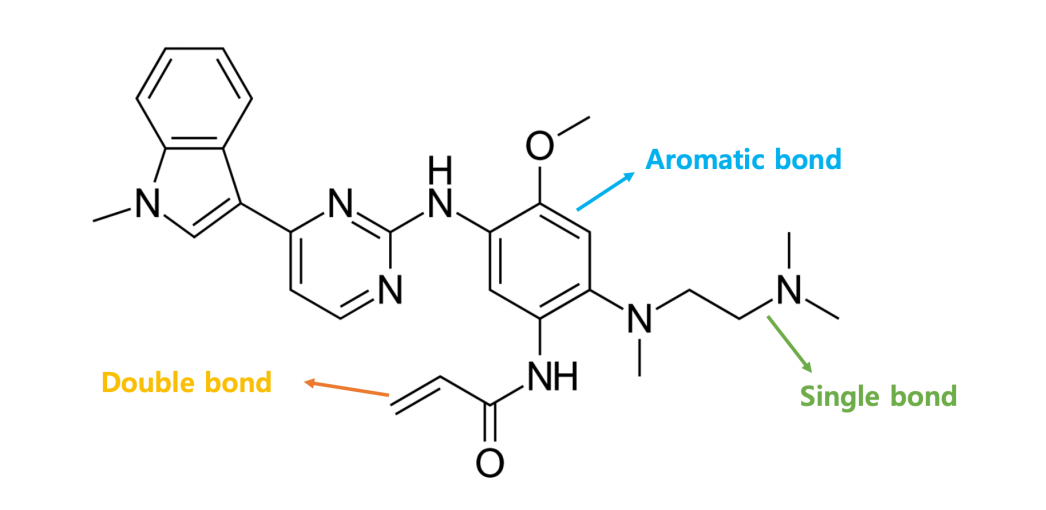A graph neural network can learn the inductive biases present in the system by not only producing the features of the elements of a system but understanding the interactions among them as well. The capability of learning of the inductive biases from a system makes it suitable for problems like few-shot learning, self-supervised or zero-shot learning.

We can use GNNs for purposes like node prediction, link prediction, finding embeddings for node, subgraph or graph etc. Now let us talk about graph convolutions.

## Graph Convolutional Networks

So in a graph problem, we are given a graph G(V, E) and the goal is to learn the node and edge representations. For each vertex i, we need to have a feature vector**Vᵢ** and the relationship between nodes can be represented using an adjacency matrix A.

GCN can be represented using a simple neural network whose mathematical representation is as follows: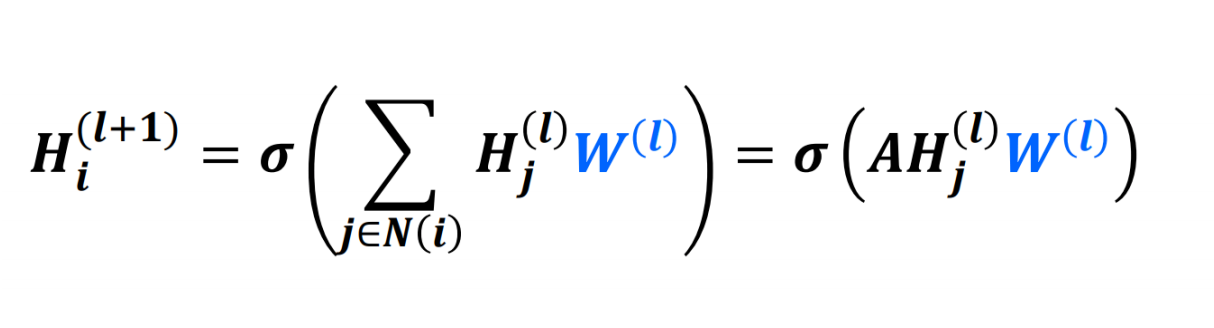where **_Hᵢˡ _**is the feature vector of node i in the lᵗʰ layer,  is the weight matrix used for the _lᵗʰ _ layer and **_N(i) _**is the set of nodes in the neighbourhood of node i. Note that Hᵢ⁰=Vᵢ

Now as you can see the weights are shared for all the nodes in a given layer akin to conventional convolutional filters and the feature vector of a node is computed upon performing some mathematical operation(here weighted sum using the edge parameter) on its neighbourhood nodes(which also is similar to that of a CNN), we come up with the term Graph Convolutional Network and  can be called as a filter in layer _ l._

As it is evident, we see two issues here, the first being that while computing the feature vector for a node, we do not consider its own feature vector unless a self-loop is there. Secondly, the adjacency matrix used here is not a normalised one, so it can cause scaling problem or gradient explosion due to the large values of the edge parameters.

To solve the first issue a self-loop is enforced and to solve the second one, a normalized form of the adjacency matrix is used which is shown below:

Self-Loop: Â =A+I

Normalization: Â = (D^(-1/2))Â(D^(-1/2))

where I is an identity matrix of shape A and **D **is a diagonal matrix whose each diagonal value correspond to the degree of the respective node in matrix Â.

In each layer, the information is passed to a node from its neighbourhood, thus the process is called Message Passing.

Now let us talk about some variants of the simple graph convolution we talked about.

#data-science #deep-learning #machine-learning #graph-neural-networks #neural-networks1593440910

## Deep learning on graphs: successes, challenges, and next steps

TL;DR This is the first in a [series of posts] where I will discuss the evolution and future trends in the field of deep learning on graphs.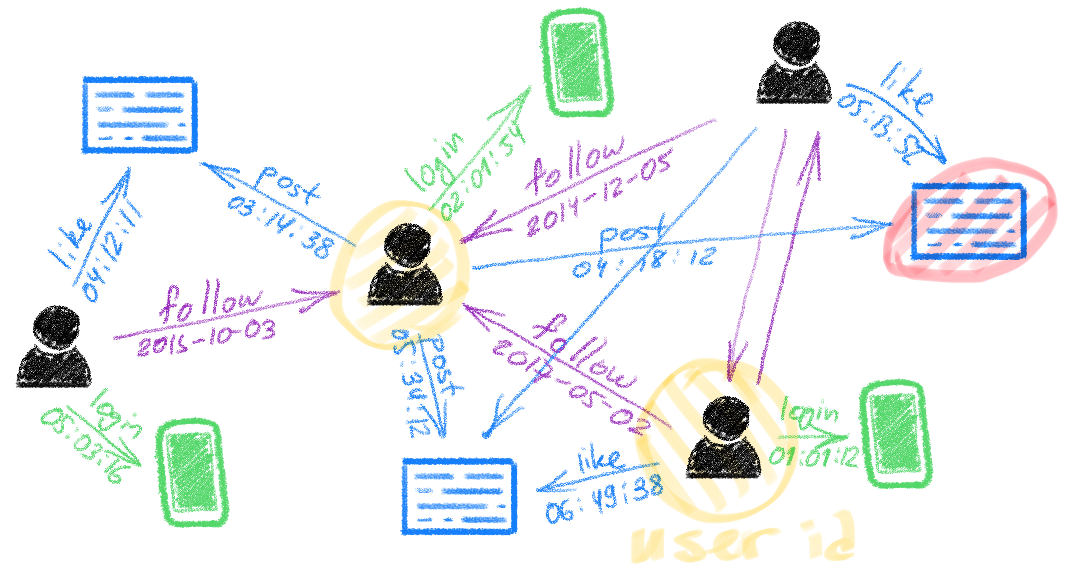Deep learning on graphs, also known as Geometric deep learning (GDL) , Graph representation learning (GRL), or relational inductive biases , has recently become one of the hottest topics in machine learning. While early works on graph learning go back at least a decade  if not two , it is undoubtedly the past few years’ progress that has taken these methods from a niche into the spotlight of the ML community and even to the popular science press (with Quanta Magazine running a series of excellent articles on geometric deep learning for the study of manifoldsdrug discovery, and protein science).

Graphs are powerful mathematical abstractions that can describe complex systems of relations and interactions in fields ranging from biology and high-energy physics to social science and economics. Since the amount of graph-structured data produced in some of these fields nowadays is enormous (prominent examples being social networks like Twitter and Facebook), it is very tempting to try to apply deep learning techniques that have been remarkably successful in other data-rich settings.

There are multiple flavours to graph learning problems that are largely application-dependent. One dichotomy is between node-wise and graph-wise problems, where in the former one tries to predict properties of individual nodes in the graph (e.g. identify malicious users in a social network), while in the latter one tries to make a prediction about the entire graph (e.g. predict solubility of a molecule). Furthermore, like in traditional ML problems, we can distinguish between supervised and unsupervised (or self-supervised) settings, as well as transductive and inductive problems.

Similarly to convolutional neural networks used in image analysis and computer vision, the key to efficient learning on graphs is designing local operations with shared weights that do message passing  between every node and its neighbours. A major difference compared to classical deep neural networks dealing with grid-structured data is that on graphs such operations are permutation-invariant, i.e. independent of the order of neighbour nodes, as there is usually no canonical way of ordering them.

Despite their promise and a series of success stories of graph representation learning (among which I can selfishly list the [acquisition by Twitter] of the graph-based fake news detection startup Fabula AI I have founded together with my students), we have not witnessed so far anything close to the smashing success convolutional networks have had in computer vision. In the following, I will try to outline my views on the possible reasons and how the field could progress in the next few years.

**Standardised benchmarks **like ImageNet were surely one of the key success factors of deep learning in computer vision, with some  even arguing that data was more important than algorithms for the deep learning revolution. We have nothing similar to ImageNet in scale and complexity in the graph learning community yet. The [Open Graph Benchmark] launched in 2019 is perhaps the first attempt toward this goal trying to introduce challenging graph learning tasks on interesting real-world graph-structured datasets. One of the hurdles is that tech companies producing diverse and rich graphs from their users’ activity are reluctant to share these data due to concerns over privacy laws such as GDPR. A notable exception is Twitter that made a dataset of 160 million tweets with corresponding user engagement graphs available to the research community under certain privacy-preserving restrictions as part of the [RecSys Challenge]. I hope that many companies will follow suit in the future.

**Software libraries **available in the public domain played a paramount role in “democratising” deep learning and making it a popular tool. If until recently, graph learning implementations were primarily a collection of poorly written and scarcely tested code, nowadays there are libraries such as [PyTorch Geometric] or [Deep Graph Library (DGL)] that are professionally written and maintained with the help of industry sponsorship. It is not uncommon to see an implementation of a new graph deep learning architecture weeks after it appears on arxiv.

Scalability is one of the key factors limiting industrial applications that often need to deal with very large graphs (think of Twitter social network with hundreds of millions of nodes and billions of edges) and low latency constraints. The academic research community has until recently almost ignored this aspect, with many models described in the literature completely inadequate for large-scale settings. Furthermore, graphics hardware (GPU), whose happy marriage with classical deep learning architectures was one of the primary forces driving their mutual success, is not necessarily the best fit for graph-structured data. In the long run, we might need specialised hardware for graphs .

**Dynamic graphs **are another aspect that is scarcely addressed in the literature. While graphs are a common way of modelling complex systems, such an abstraction is often too simplistic as real-world systems are dynamic and evolve in time. Sometimes it is the temporal behaviour that provides crucial insights about the system. Despite some recent progress, designing graph neural network models capable of efficiently dealing with continuous-time graphs represented as a stream of node- or edge-wise events is still an open research question.

#deep-learning #representation-learning #network-science #graph-neural-networks #geometric-deep-learning #deep learning